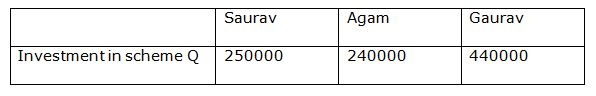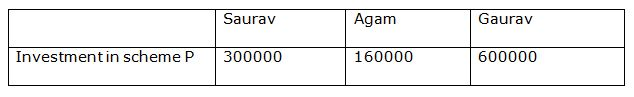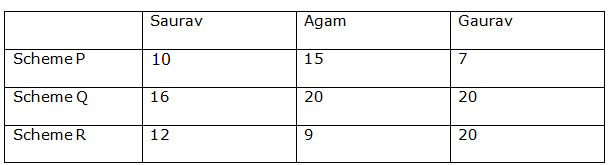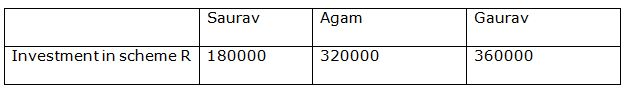# SBI PO Quantitative Aptitude Questions 2019 (Day-42) High Level New Pattern

Dear Aspirants, the most awaited notification of SBI PO – 2019 has been released. We all know that new pattern questions are introducing every year in the SBI PO exam. Further, the questions are getting tougher and beyond the level of the candidate’s expectations.

Our IBPS Guide is providing High-Level New Pattern Quantitative Aptitude Questions for SBI PO 2019 so the aspirants can practice it on a daily basis. These questions are framed by our skilled experts after understanding your needs thoroughly. Aspirants can practice these high-level questions daily to familiarize with the exact exam pattern. We wish that your rigorous preparation leads you to a successful target of becoming SBI PO.

#### “Be not afraid of growing slowly; be afraid only of standing still”

SBI PO Quantitative Aptitude Questions 2019 (Day-42) High Level New Pattern

Directions (1 – 5): Study the following information carefully and answer the question given below:

Amit can do a piece of work in 60 days. With the help of Rahul, he can complete the work in 180/7 days. Bhagat can do the same work in 15 days less than that of Rahul, when working alone. Amit started the work, Rahul and Bhagat join him every third day and thus time taken by them to complete the work is ———— (A) ————— days. Efficiency of Seema is half of the efficiency of Rahul. Amit and Bhagat started the work. Seema joined them after five days. Time taken by them to complete the remaining part of the work is ————- (B) ————- days. Seema started the work and left after 10 days. Reeta complete the remaining part of the work in 80/3 days. Amit and Reeta together can complete the work in —————– (C) —————— days. Garima and Rahul together can complete half of the work in 90/13 days. Garima and Reeta started the work and left after five days. Bhagat can complete the remaining part of the work in ————— (D) ————- days. If Reeta, Seema, Amit and Bhagat started the work together, they can complete the work in ————— (E) —————— days.

1) Find the place value of (A).

a) 23(1/11)

b) 29(3/13)

c) 37(7/17)

d) 39(1/6)

e) None of these

2) Find the place value of (B).

a) 23/2

b) 135/11

c) 133/10

d) 44/3

e) None of these

3) Find the place value of (C).

a) 15

b) 18

c) 20

d) 25

e) None of these

4) Find the place value of (D).

a) 35/2

b) 25/3

c) 18

d) 21/2

e) None of these

5) Find the place value of (E).

a) 140/11

b) 180/17

c) 160/19

d) 150/13

e) None of these

Directions (Q. 6 – 10): Saurav, Agam and Gaurav are three friends who each invested some amount in three different schemes (A, B and C). Study the data and answer the following question.

Data related to amount of investment:

Amount invested by Saurav in scheme P is half of the amount invested by Gaurav in same scheme. Agam invested Rs. 160000 in scheme P.

Ratio of investment by Saurav and Gaurav in scheme Q is in ratio 25:44. Amount invested by Agam in same scheme is 4% less than Sauravin same scheme. Gaurav invested 2 lakhs more than Agam in scheme Q. Ratio of investment by Gaurav in scheme P to scheme Q is 15:11. Agam invested double in scheme R than in scheme P.

Data related to period of investment:

Agam invested for 5 months more in scheme P than Saurav in same scheme. Gaurav invested for 7 months. Agam invested for 3 months in scheme P more than Saurav in scheme R. Ratio of time of investment by Agam in Scheme P and scheme R is 5: 3.Maximum time amount stayed invested in scheme Q and R is 20 months. Ratio of time of investment by Saurav, Agam and Gaurav in scheme Q is 4:5:5. Ratio of time of investment Saurav in scheme Q to ratio of time of investment by Gaurav in scheme R is 4:5.Saurav invested for a year in scheme R.

Data related to profit:

Profit earned by Saurav is 25% less than Agamin scheme R andAgam earned 60% less profit than Gaurav in scheme R.

6) What will be the difference of interest earned by Agam and Gaurav if they invested their amount invested in SCHEME Q offering 10% and 20% interest for 2 years compounded annually?

a) Rs. 12580

b) Rs.136850

c) Rs.143200

d) Rs.156000

e) Rs.168500

7) Quantity I: Amount invested by Saurav in scheme R is what percent of the total investment made by him in all three schemes?

Quantity II: Amount invested by Gaurav in scheme Q is what percentage of total invested made by him in all three schemes?

a) Quantity I > Quantity II

b) Quantity II > Quantity I

c) Quantity II ≥ Quantity I

d) Quantity I≥ Quantity II

e) Quantity I = Quantity II

8) What will be the profit earned by Gaurav in scheme Q if total profit is earned from scheme Q isRs.88000?

a) Rs.54000

b) Rs.66000

c) Rs.33000

d) Rs.44000

e) Rs.55000

9) What will be the interest earned by Agam from all the scheme if he invested the amount invested by him in scheme P in a scheme A giving 10% for 2 years. If amount of scheme Q in scheme B giving 15% interest for 2 years and amount of scheme R in scheme C giving 20% interest for 3 years.

a) Rs.320000

b) Rs.248500

c) Rs.296000

d) Rs.325680

e) Rs.248000

10) What is the ratio of profit earned by all three-person investingin all three schemes?

a) 283: 504: 1010

b) 204: 252: 505

c) 286: 505: 1019

d) 384: 505: 252

e) 385: 296: 465

Directions (1-5):

1/Amit = 1/60

1/Amit + 1/Rahul = 7/180

=> 1/60 + 1/Rahul = 7/180

=> 1/Rahul = 7/180 – 1/60

=> 1/Rahul = (7 – 3)/180

=> 1/Rahul = 4/180

=>1/Rahul = 1/45

1/Bhagat = 1/(45 – 15)

=>1/Bhagat = 1/30

3 days’ work = 1/60 + 1/60 + (1/60 + 1/45 + 1/30)

=>3 days’ work = (3 + 3 + 3 + 4 + 6)/180

=>3 days’ work = 19/180

=> 3 x 9 = 27 days’ work = 19/180 x 9 = 171/180

Remaining work = 1 – 171/180

= (180 – 171)/180

= 9/180

= 1/20

28 day’s work = 1/60

29 day’s work = 1/60

Remaining part of the work = 1 – 171/180 – 1/60 – 1/60

= (180 – 171 – 3 – 3)/180

= (180 – 177)/180

= 3/180

= 1/60

Let time taken by them to complete remaining part of the work = n

n x (1/60 + 1/45 + 1/30) = 1/60

=> n x (3 + 4 + 6)/180 = 1/60

=> n = 1/60 x 180/13

=> n = 3/13

Required time = 29 + 3/13 = 29(3/13)

Place value of (A) = 29(3/13)

1/Seema = ½ x 1/45

=>1/Seema = 1/90

Let time taken by them to complete remaining part of the work = n

5/60 + 5/30 + n x (1/60 + 1/30 + 1/90) = 1

=> 1/12 + 1/6 + n x (3 + 6 + 2)/180 = 1

=> 11n/180 = 1 – 1/12 – 1/6

=> 11n/180 = (12 – 1 – 2)/12

=> n = 9/12 x 180/11

=> n = 135/11

Place value of (B) =135/11

Let, Reeta alone can complete the work in n days.

10/90 + 80/3n = 1

=> 1/9 + 80/3n = 1

=> 80/3n = 1 – 1/9

=> 80/3n = (9 – 1)/9

=> n = 80/3 x 9/8

=> n = 30

1/Reeta = 1/30

Let, Amit and Reeta together can complete the work in t days.

t x (1/60 + 1/30) = 1

=> t x (1 + 2)/60 = 1

=> t = 60/3

=> t = 20 days.

Place value of (C) = 20

1/Garima + 1/Rahul = 13/90 x 1/2

=>1/Garima + 1/45 = 13/180

=> 1/Garima = 13/180 – 1/45

=> 1/Garima = (13 – 4)/180

=> 1/Garima = 9/180

=>1/Garima = 1/20

Let, required number of days = n

5/20 + 5/30 + n/30 = 1

=> ¼ + 1/6 + n/30 = 1

=> n/30 = 1 – ¼ – 1/6

=> n/30 = (12 – 3 – 2)/12

=> n = 30 x 7/12

=> n = 35/2 days

Place value of (D) = 35/2

Let, required number of days = n

n x (1/30 + 1/90 + 1/60 + 1/30) = 1

=> n x (6 + 2 + 3 + 6)/180 = 1

=> n = 180/17 days

Place value of (E) = 180/17

Directions (6 – 10):

Ratio of investment by Saurav and Gaurav in scheme Q is in ratio= 25:44

Let amount invested by Saurav in scheme Q be 25x

Amount invested by Agam in same scheme is 4% less than Saurav

Amount invested by Agam = 25x*(96/100)= 24x

Ratio of investment in scheme Q is 25x: 24x: 44x

Difference in investment by Gaurav and Agam = 20x

Given,

20x=200000

X=10,000

So investment by Saurav in scheme Q=250000

Investment by Agam in scheme Q=240000

Investment by Gaurav in scheme Q=440000Ratio of investment by Gauravin scheme p to scheme Q is 15:11

Investment by Gaurav in scheme P =440000*(15/11) =>600000

Ratio of investment of Saurav and Gaurav is scheme P is 1: 2

So, investment by Saurav in scheme P =600000*(1/2) =>300000

Investment by Agam in scheme P=160000(given)Data related to time period:

Let time of investment by Saurav in scheme P= x

So, time of investment by Agam in scheme P= x+5

Gaurav invested for 7 months (given)

Saurav invested for 12 months in scheme R (given)

Agam investment in scheme P= 12+3 =>15 months

Ratio of time of investment by Agam in scheme P and R =5: 3

Time of investment by Agam in schemeR=15*(3/5) => 9 months

Maximum time amount stayed invested in scheme Q and R is 20 months

Ratio of time of investment by Saurav, Agam and Gaurav in scheme Q Iis 4x:5x:5x

So, 5x=20

X=4=> 4x=16 months

Ratio of time of investment Saurav in scheme Q to time of investment by Gaurav in scheme R is 4:5.

Time of investment by Gaurav in scheme R =16*(5/4) => 20 months.Data related to profit

Profit=amount ×time

Amount by Sauravin R ×time/amount by Agam in scheme R × time =3/4

(Amount by Sauravin R*12)/(amount by Agam in scheme R*9) = ¾

Amount by Sauravin R/amount by Agam in scheme R=9/16

Also,

Amount by Agam in R ×time/(amount by Gaurav in scheme R × time) =2/5

(Amount by Agam in R*9)/(amount by Gaurav in scheme R*20)=2/5

Amount by Agam in R/amount by Gaurav in scheme R=8/9

Also,

Agam investment in scheme R = 2*(Agam investment in scheme P)

Agam investment in scheme R =2*(160000) => Rs.320000

Amount by Gaurav in scheme R = 320000*(9/8) =>Rs. 3,60,000

Amount by Saurav in R=320000*(9/16) => Rs. 1,80,000Agam investment in scheme Q= Rs. 240000

Gaurav investment in scheme Q= Rs.440000

Amount received by Agam compounded annually

=240000×(110/100)×(110/100)=>Rs.290400

=290400 – 240000 =>Rs.50400

Amount received by Gaurav compounded annually

=440000 ×(120/100)×(120/100)=>Rs.633600

=633600 -440000 =Rs.193600

Difference of interest = 193600-50400 =Rs.143200

Quantity I:

Amount invested by Saurav in scheme P=Rs.300000

Amount invested by Saurav in scheme Q= Rs.250000

Amount invested by Saurav in scheme R= Rs.180000

Total amount invested= 300000+250000+180000 =Rs.730000

Required percentage = (180000/730000) ×100 = 24.65%

Quantity II:

Amount invested by Gaurav in scheme P=Rs.600000

Amount invested by Gaurav in scheme Q= Rs.440000

Amount invested by Gaurav in scheme R= Rs.360000

Total amount invested= 600000+440000+360000 =Rs.1400020

Required percentage = (440000/1400020) ×100 =31.42%

Quantity II> Quantity I

Profit = Investment × time

So,

Amount by Saurav× time invested/ Amount by Agam× time invested = profit by Saurav/profit by Agam

250000 × 16/240000× 20 = 5: 6

Also,

Amount by Agam× time invested/ Amount by Gaurav× time invested = profit by Agam/profit by Gaurav

240000×20/440000×20 = 6: 11

So, required ratio = 5: 6: 11

Total profit = Rs.88000

Profit earned by Gaurav = (11/22)×88000

= >Rs.44000

Amount invested by Agam in scheme A= 160000

Amount invested by Agam in scheme B= 240000

Amount invested by Agam in scheme C= 320000

Interest earned from scheme A =160000*10*2/100 =>Rs.32000

Interest earned from scheme B =240000*15*2/100 =>Rs.72000

Interest earned from scheme C=320000*20*3/100 => Rs.192000

Total interest earned =32000+ 72000+ 192000 =>Rs. 296000

Let divide all the amounts by 1000 to make calculation easy

Profit = investment× time

Saurav = 300*10 + 250*16 +180*12 =9160

Agam = 160*15+ 240*20 +320*9 = 10080

Gaurav= 600*7+ 440*20+ 360*20= 20200

Required ratio = 204:252:505

Related Articles:

IBPSGuide Recommends Affairs Cloud Current affairs PDF